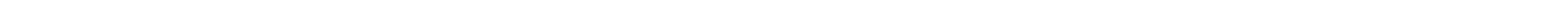In this page you will find fun examples showing how to make pretty pictures in C. You can extend the examples to making graphs and animations.

Here are a couple super-useful web pages that explains most of what I needed to know about X-Windows:
1) The X Window system (includes a short tutorial and the Xlib manual) http://tronche.com/gui/x/
2) Basic Graphics Programming With The Xlib Library - by Guy Keren http://www.silverhawk.net/notes/tutorials/x/x2.html

Refer to them when you write your own programs.

```=================== Example 1 ===================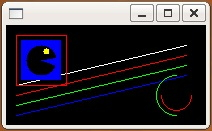//  xwindow_example.c//  This program opens a graphics window and draw four lines:  white, red, green and blue.//  Then it draws a green half-circle, and a red half-circle//  Then a red square, a blue filled square and lastly a black filled arc, and a yellow filled circle.//  This xwindow example was made possible by the helpful information and example written by//  Christophe Tronche http://tronche.com///  //  Please check out the tutorial that Mr. Tronche has posted here://  http://tronche.com/gui/x/xlib-tutorial/2nd-program-anatomy.html//  I also used information from another good tutorial which ie here://  http://user.xmission.com/~georgeps/documentation/tutorials/Xlib_Beginner.html//  Compile command:  It must use C++ because the x-window graphics library is in C++//  g++ -Wall -W -Werror xwindow_example.c -o xwindow_example -lX11// Compile command for Apple OSX only:// g++ -Wall -W -Werror xwindow_example.cpp -o xwindow_example -I/usr/X11R6/include -L/usr/X11R6/lib -lX11// (On the mac the headers amd libraries are in non-standard places.)//  After you compile it, this is the command to run it:   ("./" means "here". Linux runs whatever it finds.)//  ./xwindow_example#include <X11/Xlib.h> // Every Xlib program must include this#include <assert.h>   // I include this to test return values the lazy way#include <unistd.h>   // So we got the profile for 10 seconds#include <stdio.h>           // This has the keyboard and file IO functions#define NIL (0)       // A name for the void pointerint main(){  char keyboard_input;  GC gc;   //This will be the "graphics context"  It remembers the colors and window and various details.  XColor black_col,white_col,red_col,green_col,blue_col,yellow_col;  Colormap colormap;  char black_bits[] = "#000000";  char white_bits[] = "#FFFFFF";  char red_bits[] = "#FF0000";  char green_bits[] = "#00FF00";  char blue_bits[] = "#0000FF";  char yellow_bits[] = "#FFFF00";  Display *dpy = XOpenDisplay(NIL); assert(dpy);   // Open the display  // Get the only two predefined colors in xwindows:  Black and White  int blackColor = BlackPixel(dpy, DefaultScreen(dpy));  //int whiteColor = WhitePixel(dpy, DefaultScreen(dpy));  // Define the colors we want to use  colormap = DefaultColormap(dpy, 0);  XParseColor(dpy, colormap, black_bits, &black_col); XAllocColor(dpy, colormap, &black_col);  XParseColor(dpy, colormap, white_bits, &white_col); XAllocColor(dpy, colormap, &white_col);  XParseColor(dpy, colormap, red_bits, &red_col); XAllocColor(dpy, colormap, &red_col);  XParseColor(dpy, colormap, green_bits, &green_col);XAllocColor(dpy, colormap, &green_col);  XParseColor(dpy, colormap, blue_bits, &blue_col);XAllocColor(dpy, colormap, &blue_col);  XParseColor(dpy, colormap, yellow_bits, &yellow_col);XAllocColor(dpy, colormap, &yellow_col);  // Create the window  The numbers are the x and y locations on the screen, the width and height,   // border width (which is usually zero)  Window w = XCreateSimpleWindow(dpy, DefaultRootWindow(dpy), 0, 0, 200, 100, 0, blackColor, blackColor);  XSelectInput(dpy, w, StructureNotifyMask);        // We want to get MapNotify events  XMapWindow(dpy, w);         // "Map" the window (that is, make it appear on the screen)  for(;;){XEvent e; XNextEvent(dpy,&e); if(e.type == MapNotify) break;} //Wait for the MapNotify event  // which means that the window has appeared on the screen.  gc = XCreateGC(dpy, w, 0, NIL);        // Create a "Graphics Context"    //  We are finally ready to do some drawing!  Whew!  //  Lets try XDrawLine(display, d, gc, x1, y1, x2, y2)  XSetForeground(dpy, gc, red_col.pixel);  // Tell the GC we draw using the RED color  XDrawLine(dpy, w, gc, 10, 70, 180, 30);      // Draw theline  XSetForeground(dpy, gc, green_col.pixel);  // Tell the GC we draw using the GREEN color  XDrawLine(dpy, w, gc, 10, 80, 180, 40);      // Draw the line  XSetForeground(dpy, gc, blue_col.pixel);  // Tell the GC we draw using the BLUE color  XDrawLine(dpy, w, gc, 10, 90, 180, 50);      // Draw the line  //XSetForeground(dpy, gc, whiteColor);  // Tell the GC we draw using the WHITE color (Method 1)  XSetForeground(dpy, gc, white_col.pixel);  // Tell the GC we draw using the WHITE color (Method 2)  XDrawLine(dpy, w, gc, 10, 60, 180, 20);      // Draw the line  //  Lets try XDrawArc(display, d, gc, x, y, width, height, angle1, angle2)  //  The angle are in weird units of 1/64th of a degree.  angle1 is the start angle. (Zero is to the right.)   //  angle2 is how much of an arc to make.  XSetForeground(dpy, gc, green_col.pixel);  // Tell the GC we draw using the GREEN color  XDrawArc(dpy, w, gc, 150, 50, 40, 40, 90*64, 180*64);      // Draw the arc  XSetForeground(dpy, gc, red_col.pixel);  // Tell the GC we draw using the RED color  XDrawArc(dpy, w, gc, 155, 55, 30, 30, 180*64, 180*64);      // Draw the arc  //  Lets try XDrawRectangle(display, d, gc, x, y, width, height)  XSetForeground(dpy, gc, red_col.pixel);  // Tell the GC we draw using the RED color  XDrawRectangle(dpy, w, gc, 10, 10, 50, 50);      // Draw the rectangle  //  Lets try XFillRectangle(display, d, gc, x, y, width, height)  XSetForeground(dpy, gc, blue_col.pixel);  // Tell the GC we draw using the BLUE color  XFillRectangle(dpy, w, gc, 15, 15, 40, 40);      // Draw the filled rectangle  //  Lets try XFillArc(display, d, gc,  x, y, width, height, angle1, angle2)  //  The angle are in weird units of 1/64th of a degree.  angle1 is the start angle. (Zero is to the right.)   //  angle2 is how much of an arc to make.  XSetForeground(dpy, gc, black_col.pixel);  // Tell the GC we draw using the BLACK color  XFillArc(dpy, w, gc, 20, 20, 30, 30, 10*64, 330*64);      // Draw the filled arc  XSetForeground(dpy, gc, yellow_col.pixel);  // Tell the GC we draw using the YELLOW color  XFillArc(dpy, w, gc, 37, 23, 6, 6, 0*64, 360*64);      // Draw the filled circle  XFlush(dpy); // Tell the graphics server that it should really show us the results now.  sleep(1);  // Wait for 1 second  printf("Press enter when done.\n");  fgets (keyboard_input,100,stdin);   return(0);}=================== Example 2 ===================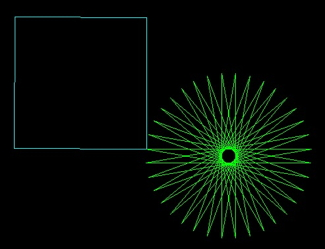//  turtle_graphics_example.c//  This program creates an easy way for students to make beautiful patterns, via the turtle graphics metaphore.//  The turtle has a pen on its tail.  As he moves, he leaves a line behind him.//  The turtle commands are forward, left, right, home, pen_up (no line), pen_down (line), //  and get_pen (select a color).//  Students: go down to the bottom of the example, and you'll see where you can create your own patterns.//  As-is, this program opens a graphics window and the turtle draws a green star and a cyan colored square.//  This xwindow example was made possible by the helpful information and example written by//  Christophe Tronche http://tronche.com///  //  Please check out the tutorial that Mr. Tronche has posted here://  http://tronche.com/gui/x/xlib-tutorial/2nd-program-anatomy.html//  I also used information from another good tutorial which ie here://  http://user.xmission.com/~georgeps/documentation/tutorials/Xlib_Beginner.html//  Compile command:  It must use C++ because the x-window graphics library is in C++//  g++ -Wall -W -Werror turtle_graphics_example.c -o turtle_graphics_example -lX11//  After you compile it, this is the command to run it:   ("./" means "here". Linux runs whatever it finds.)//  ./turtle_graphics_example#include <X11/Xlib.h> // Every Xlib program must include this#include <assert.h>   // I include this to test return values the lazy way#include <unistd.h>   // So we got the profile for 10 seconds#include <stdio.h>    // This has the keyboard and file IO functions#include <math.h>     // sine, cosine and various math functions#include <stdbool.h>  // Defines true, false#define NIL (0)       // A name for the void pointer#define window_width 800#define window_height 600#define origin_x 400#define origin_y 300GC gc;   //This will be the "graphics context"  It remembers the colors and window and various details.Display *dpy;Window w;double turtle_x; double turtle_y; double turtle_heading;  //heading is in degrees.  zero is to the right.bool turtle_pen_active = true;int home() {  turtle_x=0.0; turtle_y=0.0; turtle_heading=0.0;  return(0);}int forward(double distance) {  double new_x=turtle_x+distance*cos(turtle_heading*M_PI/180.0);  double new_y=turtle_y+distance*sin(turtle_heading*M_PI/180.0);  int x1=turtle_x+origin_x;  int y1=window_height-(turtle_y+origin_y);  int x2=new_x+origin_x;  int y2=window_height-(new_y+origin_y);  if (turtle_pen_active) XDrawLine(dpy, w, gc, x1, y1, x2, y2);      // Draw theline  turtle_x=new_x;turtle_y=new_y;  return(0);}int right(double angle) { turtle_heading = turtle_heading-angle; return (0); }int left(double angle) { turtle_heading = turtle_heading+angle; return (0); }int get_pen(XColor desired_col) {  XSetForeground(dpy, gc, desired_col.pixel);  // Tell the GC we draw using the desired color  return(0);}int pen_up() {turtle_pen_active = false; return(0); }int pen_down() {turtle_pen_active = true; return(0); }int main(){  char keyboard_input;  XColor black_col,white_col,red_col,green_col,blue_col,yellow_col,magenta_col,cyan_col;  Colormap colormap;  char black_bits[] = "#000000";  char white_bits[] = "#FFFFFF";    // Mix red, green and blue to get white  char red_bits[] = "#FF0000";  char green_bits[] = "#00FF00";  char blue_bits[] = "#0000FF";  char yellow_bits[] = "#FFFF00";   // Mix red and green to get yellow  char magenta_bits[] = "#FF00FF";  // A sort of purple color  char cyan_bits[] = "#00FFFF";     // A blue-green color  //Display *dpy = XOpenDisplay(NIL);   dpy = XOpenDisplay(NIL); assert(dpy);   // Open the display  // Define the colors we want to use  colormap = DefaultColormap(dpy, 0);  XParseColor(dpy, colormap, black_bits, &black_col); XAllocColor(dpy, colormap, &black_col);  XParseColor(dpy, colormap, white_bits, &white_col); XAllocColor(dpy, colormap, &white_col);  XParseColor(dpy, colormap, red_bits, &red_col); XAllocColor(dpy, colormap, &red_col);  XParseColor(dpy, colormap, green_bits, &green_col);XAllocColor(dpy, colormap, &green_col);  XParseColor(dpy, colormap, blue_bits, &blue_col);XAllocColor(dpy, colormap, &blue_col);  XParseColor(dpy, colormap, yellow_bits, &yellow_col);XAllocColor(dpy, colormap, &yellow_col);  XParseColor(dpy, colormap, magenta_bits, &magenta_col);XAllocColor(dpy, colormap, &magenta_col);  XParseColor(dpy, colormap, cyan_bits, &cyan_col);XAllocColor(dpy, colormap, &cyan_col);  // Create the window  The numbers are the x and y locations on the screen, the width and height,   // border width (which is usually zero)  w = XCreateSimpleWindow(dpy, DefaultRootWindow(dpy), 0, 0, window_width, window_height,  0, black_col.pixel, black_col.pixel);  XSelectInput(dpy, w, StructureNotifyMask);        // We want to get MapNotify events  XMapWindow(dpy, w);         // "Map" the window (that is, make it appear on the screen)  for(;;){XEvent e; XNextEvent(dpy,&e); if(e.type == MapNotify) break;} //Wait for the MapNotify event  // which means that the window has appeared on the screen.  gc = XCreateGC(dpy, w, 0, NIL);        // Create a "Graphics Context"  get_pen(white_col);  //  We are finally ready to do some drawing!  Whew!    // ................  Students: you put your beautiful code HERE: .......................  int i;  home();      // Send the turtle to the middle of the window.  That's its "home"  get_pen(green_col);  for (i=0; i<36; i++) {    forward(250);    //printf("1 x=%g y=%g heading=%g\n",turtle_x,turtle_y,turtle_heading); //Optional: use for debugging    right(170);    //usleep(100000); XFlush(dpy); // Optional: use this to see the lines being drawn, one-by-one  }  pen_up(); home();   left(90); pen_down();  for (i=0; i<4; i++) {    get_pen(cyan_col);    forward(200);    //printf("2 x=%g y=%g heading=%g\n",turtle_x,turtle_y,turtle_heading); //Optional: use for debugging    left(90);  }  XFlush(dpy); // Tell the graphics server that it should really show us the results now.  sleep(1);  // Wait for 1 second  printf("Press enter when done.\n");  fgets (keyboard_input,100,stdin);   return(0);}=================== Example 3 ===================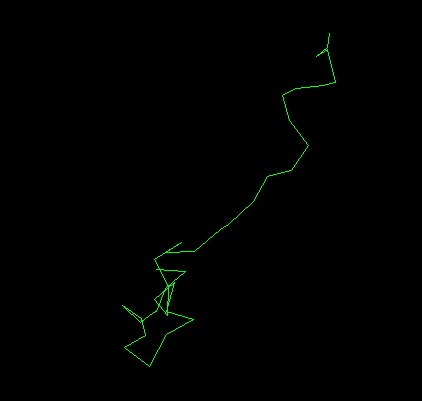//  drunken_turtle.c//  This example introduces a new turtle command:  gotoxy//  Take a look at how it works.  You will see that creating new commands is pretty easy.//  This example also introduces random numbers and how to create them.  Note that computers can't//  make truly random numbers, so the time of the program's start is used as a random starting point//  for a pseudo-random sequence.   Its random enough that you'll never know the difference.//  This xwindow example was made possible by the helpful information and example written by//  Christophe Tronche http://tronche.com///  Please check out the tutorial that Mr. Tronche has posted here://  http://tronche.com/gui/x/xlib-tutorial/2nd-program-anatomy.html//  I also used information from another good tutorial which ie here://  http://user.xmission.com/~georgeps/documentation/tutorials/Xlib_Beginner.html//  Compile command:  It must use C++ because the x-window graphics library is in C++//  g++ -Wall -W -Werror drunken_turtle.c -o drunken_turtle -lX11//  After you compile it, this is the command to run it:   ("./" means "here". Linux runs whatever it finds.)//  ./drunken_turtle#include <X11/Xlib.h> // Every Xlib program must include this#include <assert.h>   // I include this to test return values the lazy way#include <unistd.h>   // So we got the profile for 10 seconds#include <stdio.h>    // This has the keyboard and file IO functions#include <math.h>     // sine, cosine and various math functions#include <stdbool.h>  // Defines true, false#include <time.h>     // I need this because I will use the time to "seed" the random function#include <stdlib.h>   // I need this because it has the random functions#define NIL (0)       // A name for the void pointer#define window_width 800#define window_height 600#define origin_x 400#define origin_y 300double rand_range(double fMin, double fMax){    double f = (double)rand() / RAND_MAX;    return fMin + f * (fMax - fMin);}GC gc;   //This will be the "graphics context"  It remembers the colors and window and various details.Display *dpy;Window w;double turtle_x; double turtle_y; double turtle_heading;  //heading is in degrees.  zero is to the right.bool turtle_pen_active = true;int home() {  turtle_x=0.0; turtle_y=0.0; turtle_heading=0.0;  return(0);}int gotoxy (double new_x, double new_y) {  int x1=turtle_x+origin_x;  int y1=window_height-(turtle_y+origin_y);  int x2=new_x+origin_x;  int y2=window_height-(new_y+origin_y);  if (turtle_pen_active) XDrawLine(dpy, w, gc, x1, y1, x2, y2);      // Draw theline  turtle_x=new_x;turtle_y=new_y;  return(0);}int forward(double distance) {  double new_x=turtle_x+distance*cos(turtle_heading*M_PI/180.0);  double new_y=turtle_y+distance*sin(turtle_heading*M_PI/180.0);  int x1=turtle_x+origin_x;  int y1=window_height-(turtle_y+origin_y);  int x2=new_x+origin_x;  int y2=window_height-(new_y+origin_y);  if (turtle_pen_active) XDrawLine(dpy, w, gc, x1, y1, x2, y2);      // Draw theline  turtle_x=new_x;turtle_y=new_y;  return(0);}int right(double angle) { turtle_heading = turtle_heading-angle; return (0); }int left(double angle) { turtle_heading = turtle_heading+angle; return (0); }int get_pen(XColor desired_col) {  XSetForeground(dpy, gc, desired_col.pixel);  // Tell the GC we draw using the desired color  return(0);}int pen_up() {turtle_pen_active = false; return(0); }int pen_down() {turtle_pen_active = true; return(0); }int main(){  char keyboard_input;  XColor black_col,white_col,red_col,green_col,blue_col,yellow_col,magenta_col,cyan_col;  Colormap colormap;  char black_bits[] = "#000000";  char white_bits[] = "#FFFFFF";    // Mix red, green and blue to get white  char red_bits[] = "#FF0000";  char green_bits[] = "#00FF00";  char blue_bits[] = "#0000FF";  char yellow_bits[] = "#FFFF00";   // Mix red and green to get yellow  char magenta_bits[] = "#FF00FF";  // A sort of purple color  char cyan_bits[] = "#00FFFF";     // A blue-green color  //Display *dpy = XOpenDisplay(NIL);   dpy = XOpenDisplay(NIL); assert(dpy);   // Open the display  // Define the colors we want to use  colormap = DefaultColormap(dpy, 0);  XParseColor(dpy, colormap, black_bits, &black_col); XAllocColor(dpy, colormap, &black_col);  XParseColor(dpy, colormap, white_bits, &white_col); XAllocColor(dpy, colormap, &white_col);  XParseColor(dpy, colormap, red_bits, &red_col); XAllocColor(dpy, colormap, &red_col);  XParseColor(dpy, colormap, green_bits, &green_col);XAllocColor(dpy, colormap, &green_col);  XParseColor(dpy, colormap, blue_bits, &blue_col);XAllocColor(dpy, colormap, &blue_col);  XParseColor(dpy, colormap, yellow_bits, &yellow_col);XAllocColor(dpy, colormap, &yellow_col);  XParseColor(dpy, colormap, magenta_bits, &magenta_col);XAllocColor(dpy, colormap, &magenta_col);  XParseColor(dpy, colormap, cyan_bits, &cyan_col);XAllocColor(dpy, colormap, &cyan_col);  // Create the window  The numbers are the x and y locations on the screen, the width and height,   // border width (which is usually zero)  w = XCreateSimpleWindow(dpy, DefaultRootWindow(dpy), 0, 0, window_width, window_height,  0, black_col.pixel, black_col.pixel);  XSelectInput(dpy, w, StructureNotifyMask);        // We want to get MapNotify events  XMapWindow(dpy, w);         // "Map" the window (that is, make it appear on the screen)  for(;;){XEvent e; XNextEvent(dpy,&e); if(e.type == MapNotify) break;} //Wait for the MapNotify event  // which means that the window has appeared on the screen.  gc = XCreateGC(dpy, w, 0, NIL);        // Create a "Graphics Context"  get_pen(white_col);  //  We are finally ready to do some drawing!  Whew!    // ................  Students: you put your beautiful code HERE: .......................  srand(time(NULL)); //  Get ready to make random numbers.  The time "seeds" the generator.  int i;  double x=0,  y=0;  home();      // Send the turtle to the middle of the window.  That's its "home"  get_pen(green_col);  pen_down();  for (i=0; i<36; i++) {    x = x + rand_range(-30.0, 30.0);  //Generates random number between these two numbers.    y = y + rand_range(-30.0, 30.0)+5.0;    gotoxy(x, y);   // Move the turtle to the new location.  It will draw a line if the pen is down.    XFlush(dpy); // Tell the graphics server that it should really show us the results now.    printf("1 x=%g y=%g heading=%g\n",turtle_x,turtle_y,turtle_heading); //Optional: use for debugging    usleep(100000); XFlush(dpy); // Optional: use this to see the lines being drawn, one-by-one  }  XFlush(dpy); // Tell the graphics server that it should really show us the results now.  sleep(1);  // Wait for 1 second  printf("Press enter when done.\n");  fgets (keyboard_input,100,stdin);   return(0);}=================== Example 4 ===================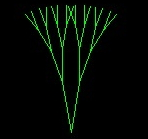//  turtle_tree.c//  This example introduces RECURSION.  This means that a function calls itself.//  Imagine you could instantly create a copy of yourself.  You could tell copy 1 to do your math homework//  and copy 2 to do your english homework.//  Then copy 1 copies itself.  It tells copy 1-1 to do all the even math problems and copy 1-2 to do all the//  odd math problems and on and on.//  Soon you have hundreds of copies of yourself and the homework gets done really really fast!   Wow!//  In this example, "branch" draws a couple sticks.  But it wants more sticks at the end of each stick.//  So it copies itself and each copy draws more sticks!  You'll see...//  Compile command:  It must use C++ because the x-window graphics library is in C++//  g++ -Wall -W -Werror turtle_tree.c -o turtle_tree -lX11//  After you compile it, this is the command to run it:   ("./" means "here". Linux runs whatever it finds.)//  ./turtle_tree#include <X11/Xlib.h> // Every Xlib program must include this#include <assert.h>   // I include this to test return values the lazy way#include <unistd.h>   // So we got the profile for 10 seconds#include <stdio.h>    // This has the keyboard and file IO functions#include <math.h>     // sine, cosine and various math functions#include <stdbool.h>  // Defines true, false#include <time.h>     // I need this because I will use the time to "seed" the random function#include <stdlib.h>   // I need this because it has the random functions#define NIL (0)       // A name for the void pointer#define window_width 800#define window_height 600#define origin_x 400#define origin_y 300double rand_range(double fMin, double fMax){    double f = (double)rand() / RAND_MAX;    return fMin + f * (fMax - fMin);}GC gc;   //This will be the "graphics context"  It remembers the colors and window and various details.Display *dpy;Window w;double turtle_x; double turtle_y; double turtle_heading;  //heading is in degrees.  zero is to the right.bool turtle_pen_active = true;int home() {  turtle_x=0.0; turtle_y=0.0; turtle_heading=0.0;  return(0);}int gotoxy (double new_x, double new_y) {  int x1=turtle_x+origin_x;  int y1=window_height-(turtle_y+origin_y);  int x2=new_x+origin_x;  int y2=window_height-(new_y+origin_y);  if (turtle_pen_active) XDrawLine(dpy, w, gc, x1, y1, x2, y2);      // Draw theline  turtle_x=new_x;turtle_y=new_y;  return(0);}int forward(double distance) {  double new_x=turtle_x+distance*cos(turtle_heading*M_PI/180.0);  double new_y=turtle_y+distance*sin(turtle_heading*M_PI/180.0);  int x1=turtle_x+origin_x;  int y1=window_height-(turtle_y+origin_y);  int x2=new_x+origin_x;  int y2=window_height-(new_y+origin_y);  if (turtle_pen_active) XDrawLine(dpy, w, gc, x1, y1, x2, y2);      // Draw theline  turtle_x=new_x;turtle_y=new_y;  return(0);}int right(double angle) { turtle_heading = turtle_heading-angle; return (0); }int left(double angle) { turtle_heading = turtle_heading+angle; return (0); }int get_pen(XColor desired_col) {  XSetForeground(dpy, gc, desired_col.pixel);  // Tell the GC we draw using the desired color  return(0);}int pen_up() {turtle_pen_active = false; return(0); }int pen_down() {turtle_pen_active = true; return(0); }//======================================================================================================int branch(int depth)   //  This is a RECURSIVE dunction that will make a FRACTAL{  printf("depth=%d\n",depth);  if (depth > 4) return(0);   //  The is the recursion ESCAPE route.  Without it the program crashes.  depth++;  left(10); forward(100/depth); branch(depth);  // branch is going to branch!  How weird!  //usleep(100000); XFlush(dpy); // Optional: use this to see the lines being drawn, one-by-one  forward(-100/depth);  right(20); forward(100/depth); branch(depth); // branch will branch again!  Weirder!  //usleep(100000); XFlush(dpy); // Optional: use this to see the lines being drawn, one-by-one  forward(-100/depth);  left(10);  return(0);}//======================================================================================================int main(){  char keyboard_input;  XColor black_col,white_col,red_col,green_col,blue_col,yellow_col,magenta_col,cyan_col;  Colormap colormap;  char black_bits[] = "#000000";  char white_bits[] = "#FFFFFF";    // Mix red, green and blue to get white  char red_bits[] = "#FF0000";  char green_bits[] = "#00FF00";  char blue_bits[] = "#0000FF";  char yellow_bits[] = "#FFFF00";   // Mix red and green to get yellow  char magenta_bits[] = "#FF00FF";  // A sort of purple color  char cyan_bits[] = "#00FFFF";     // A blue-green color  //Display *dpy = XOpenDisplay(NIL);   dpy = XOpenDisplay(NIL); assert(dpy);   // Open the display  // Define the colors we want to use  colormap = DefaultColormap(dpy, 0);  XParseColor(dpy, colormap, black_bits, &black_col); XAllocColor(dpy, colormap, &black_col);  XParseColor(dpy, colormap, white_bits, &white_col); XAllocColor(dpy, colormap, &white_col);  XParseColor(dpy, colormap, red_bits, &red_col); XAllocColor(dpy, colormap, &red_col);  XParseColor(dpy, colormap, green_bits, &green_col);XAllocColor(dpy, colormap, &green_col);  XParseColor(dpy, colormap, blue_bits, &blue_col);XAllocColor(dpy, colormap, &blue_col);  XParseColor(dpy, colormap, yellow_bits, &yellow_col);XAllocColor(dpy, colormap, &yellow_col);  XParseColor(dpy, colormap, magenta_bits, &magenta_col);XAllocColor(dpy, colormap, &magenta_col);  XParseColor(dpy, colormap, cyan_bits, &cyan_col);XAllocColor(dpy, colormap, &cyan_col);  // Create the window  The numbers are the x and y locations on the screen, the width and height,   // border width (which is usually zero)  w = XCreateSimpleWindow(dpy, DefaultRootWindow(dpy), 0, 0, window_width, window_height,  0, black_col.pixel, black_col.pixel);  XSelectInput(dpy, w, StructureNotifyMask);        // We want to get MapNotify events  XMapWindow(dpy, w);         // "Map" the window (that is, make it appear on the screen)  for(;;){XEvent e; XNextEvent(dpy,&e); if(e.type == MapNotify) break;} //Wait for the MapNotify event  // which means that the window has appeared on the screen.  gc = XCreateGC(dpy, w, 0, NIL);        // Create a "Graphics Context"  get_pen(white_col);  //  We are finally ready to do some drawing!  Whew!    // ................  Students: you put your beautiful code HERE: .......................  home();      // Send the turtle to the middle of the window.  That's its "home"  get_pen(green_col);  pen_down();  left(90);  branch(1);    //Draw the FRACTAL  XFlush(dpy); // Tell the graphics server to show us the results now.  sleep(1);  // Wait for 1 second  printf("Press enter when done.\n");  fgets (keyboard_input,100,stdin);   return(0);}=================== Example 5 ===================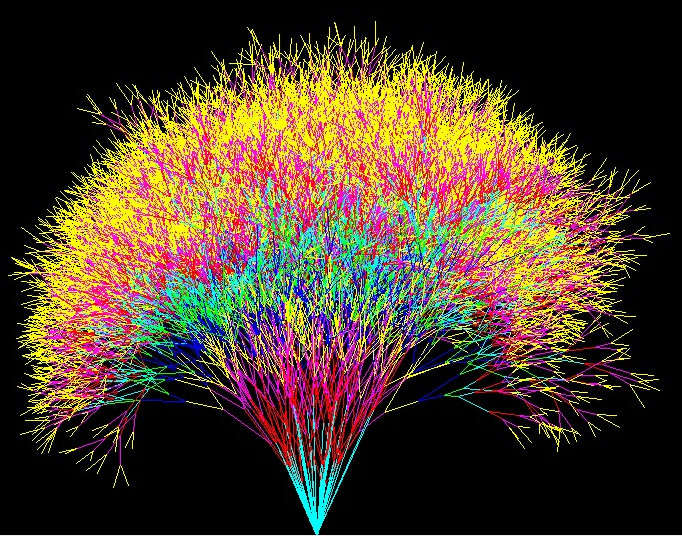//  turtle_tree2.c//  This example introduces RECURSION.  This means that a function calls itself.//  Imagine you could instantly create a copy of yourself.  You could tell copy 1 to do your math homework//  and copy 2 to do your english homework.//  Then copy 1 copies itself.  It tells copy 1-1 to do all the even math problems and copy 1-2 to do all the//  odd math problems and on and on.//  Soon you have hundreds of copies of yourself and the homework gets done really really fast!   Wow!//  In this example, "branch" draws a couple sticks.  But it wants more sticks at the end of each stick.//  So it copies itself and each copy draws more sticks!  You'll see...//  Compile command:  It must use C++ because the x-window graphics library is in C++//  g++ -Wall -W -Werror turtle_tree2.c -o turtle_tree2 -lX11//  After you compile it, this is the command to run it:   ("./" means "here". Linux runs whatever it finds.)//  ./turtle_tree2#include <X11/Xlib.h> // Every Xlib program must include this#include <assert.h>   // I include this to test return values the lazy way#include <unistd.h>   // So we got the profile for 10 seconds#include <stdio.h>    // This has the keyboard and file IO functions#include <math.h>     // sine, cosine and various math functions#include <stdbool.h>  // Defines true, false#include <time.h>     // I need this because I will use the time to "seed" the random function#include <stdlib.h>   // I need this because it has the random functions#define NIL (0)       // A name for the void pointer#define window_width 800#define window_height 600#define origin_x 400#define origin_y 300double rand_range(double fMin, double fMax){    double f = (double)rand() / RAND_MAX;    return fMin + f * (fMax - fMin);}GC gc;   //This will be the "graphics context"  It remembers the colors and window and various details.Display *dpy;Window w;double turtle_x; double turtle_y; double turtle_heading;  //heading is in degrees.  zero is to the right.bool turtle_pen_active = true;int home() {  turtle_x=0.0; turtle_y=0.0; turtle_heading=0.0;  return(0);}int gotoxy (double new_x, double new_y) {  int x1=turtle_x+origin_x;  int y1=window_height-(turtle_y+origin_y);  int x2=new_x+origin_x;  int y2=window_height-(new_y+origin_y);  if (turtle_pen_active) XDrawLine(dpy, w, gc, x1, y1, x2, y2);      // Draw theline  turtle_x=new_x;turtle_y=new_y;  return(0);}int forward(double distance) {  double new_x=turtle_x+distance*cos(turtle_heading*M_PI/180.0);  double new_y=turtle_y+distance*sin(turtle_heading*M_PI/180.0);  int x1=turtle_x+origin_x;  int y1=window_height-(turtle_y+origin_y);  int x2=new_x+origin_x;  int y2=window_height-(new_y+origin_y);  if (turtle_pen_active) XDrawLine(dpy, w, gc, x1, y1, x2, y2);      // Draw theline  turtle_x=new_x;turtle_y=new_y;  return(0);}int right(double angle) { turtle_heading = turtle_heading-angle; return (0); }int left(double angle) { turtle_heading = turtle_heading+angle; return (0); }XColor black_col,white_col,red_col,green_col,blue_col,yellow_col,magenta_col,cyan_col;int get_pen(XColor desired_col) {  XSetForeground(dpy, gc, desired_col.pixel);  // Tell the GC we draw using the desired color  return(0);}int get_pen_n(int pen_number) {  switch (pen_number) {    case 0: get_pen(black_col); break;    case 1: get_pen(blue_col); break;    case 2: get_pen(green_col); break;    case 3: get_pen(cyan_col); break;    case 4: get_pen(red_col); break;    case 5: get_pen(magenta_col); break;    case 6: get_pen(yellow_col); break;    case 7: get_pen(white_col); break;  }  return(0);}int pen_up() {turtle_pen_active = false; return(0); }int pen_down() {turtle_pen_active = true; return(0); }//======================================================================================================int branch(int depth)   //  This is a RECURSIVE dunction that will make a FRACTAL{  double branch_length, branch_angle;  printf("depth=%d\n",depth);  if (depth > 10) return(0);   //  The is the recursion ESCAPE route.  Without it the program crashes.  depth++;  get_pen_n(depth%6+1);  branch_length=rand_range(100,300)/depth;  branch_angle=rand_range(1,25);  left(branch_angle); forward(branch_length); branch(depth);  // branch is going to branch!  How weird!  //usleep(100000); XFlush(dpy); // Optional: use this to see the lines being drawn, one-by-one  forward(-branch_length); right(branch_angle);  branch_length=rand_range(100,300)/depth;  branch_angle=rand_range(1,25);  right(branch_angle); forward(branch_length); branch(depth); // branch will branch again!  Weirder!  //usleep(100000); XFlush(dpy); // Optional: use this to see the lines being drawn, one-by-one  forward(-branch_length);  left(branch_angle);  get_pen_n((depth-1)%6+1);  return(0);}//======================================================================================================int main(){  char keyboard_input;  Colormap colormap;  char black_bits[] = "#000000";  char white_bits[] = "#FFFFFF";    // Mix red, green and blue to get white  char red_bits[] = "#FF0000";  char green_bits[] = "#00FF00";  char blue_bits[] = "#0000FF";  char yellow_bits[] = "#FFFF00";   // Mix red and green to get yellow  char magenta_bits[] = "#FF00FF";  // A sort of purple color  char cyan_bits[] = "#00FFFF";     // A blue-green color  //Display *dpy = XOpenDisplay(NIL);   dpy = XOpenDisplay(NIL); assert(dpy);   // Open the display  // Define the colors we want to use  colormap = DefaultColormap(dpy, 0);  XParseColor(dpy, colormap, black_bits, &black_col); XAllocColor(dpy, colormap, &black_col);  XParseColor(dpy, colormap, white_bits, &white_col); XAllocColor(dpy, colormap, &white_col);  XParseColor(dpy, colormap, red_bits, &red_col); XAllocColor(dpy, colormap, &red_col);  XParseColor(dpy, colormap, green_bits, &green_col);XAllocColor(dpy, colormap, &green_col);  XParseColor(dpy, colormap, blue_bits, &blue_col);XAllocColor(dpy, colormap, &blue_col);  XParseColor(dpy, colormap, yellow_bits, &yellow_col);XAllocColor(dpy, colormap, &yellow_col);  XParseColor(dpy, colormap, magenta_bits, &magenta_col);XAllocColor(dpy, colormap, &magenta_col);  XParseColor(dpy, colormap, cyan_bits, &cyan_col);XAllocColor(dpy, colormap, &cyan_col);  // Create the window  The numbers are the x and y locations on the screen, the width and height,   // border width (which is usually zero)  w = XCreateSimpleWindow(dpy, DefaultRootWindow(dpy), 0, 0, window_width, window_height,  0, black_col.pixel, black_col.pixel);  XSelectInput(dpy, w, StructureNotifyMask);        // We want to get MapNotify events  XMapWindow(dpy, w);         // "Map" the window (that is, make it appear on the screen)  for(;;){XEvent e; XNextEvent(dpy,&e); if(e.type == MapNotify) break;} //Wait for the MapNotify event  // which means that the window has appeared on the screen.  gc = XCreateGC(dpy, w, 0, NIL);        // Create a "Graphics Context"  get_pen(white_col);  //  We are finally ready to do some drawing!  Whew!    // ................  Students: you put your beautiful code HERE: .......................  srand(time(NULL)); //  Get ready to make random numbers.  The time "seeds" the generator.  int i;  for (i=0; i< 20; i++) {    home();      // Send the turtle to the middle of the window.  That's its "home"    get_pen(green_col);  pen_up();    left(90);    forward(-300);  pen_down();    branch(1);    //Draw the FRACTAL    XFlush(dpy); // Tell the graphics server to show us the results now.    usleep(100000);  // Wait for 100000 micro seconds  }  printf("Press enter when done.\n");  fgets (keyboard_input,100,stdin);   return(0);}
```